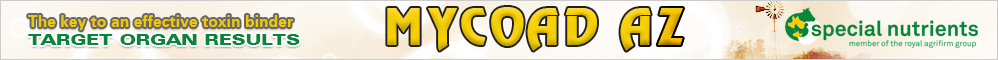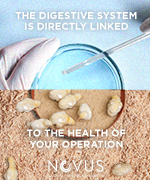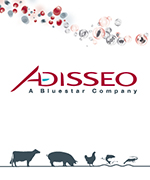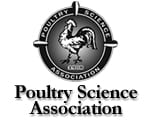# Economics of Poultry Feeding: From least cost to maximum profit feed formulation

Published on: 7/20/2020
Author/s :

The feeding program for poultry has a major influence on profitability as feed cost accounts for up to 75% of production cost. Conventionally, it is assumed that requirements are either listed in tables or breeder nutrition guides. The Requirement Approach stems from the broken line, which is a simplification of bird response and implies that at different levels of energy or nutrients, efficiency does not change or it is zero. Bird response is curvilinear and this implies that diminishing returns operate and for that reason, economic analysis has to be used to make decisions as to the optimum level of energy or nutrients. The Response Approach necessarily assumes that growth or production rate  and feed intake, that is to say,  bird performance, has to be known to determine the optimum level.

The economic analysis necessary to optimize production has included from feed cost, going through feeding cost per unit of gain up to income over feed cost or margin as profit indicators (income – fixed cost – variable cost). Income over feed cost or margin can be mathematically represented by the profit equation:

π = Py*Y – Px*X

where:

π = income over feed cost or margin over cost

Py = Meat or egg price per kilo

Y = Live weight or egg mass, kg

Px = Feed Price per kilo

X = Feed intake

Least cost feed formulation has consolidated feed cost as a profit indicator that assumes that performance is constant and independent of the nutritional program. However, it is widely known that changes in energy, nutrient or ingredient level often change bird performance and feed cost is not appropriate to evaluate the economics of poultry feeding. As it is shown in the profit equation, feed cost (Px) is just a part of the equation.

Manipulating the profit equation and dividing by Y we have profit per kilo

π/Y = Py – Px *(X/Y)

where:

(X/Y) = feed conversion

Feed cost per unit of gain (Px*(X/Y)) is an improvement but can only be used when, on changing the nutritional program, changes in feed conversion are expected with no change in bird growth or production rate.

Therefore, income over feed cost or margin is the best method to determine the economic value of a nutritional program, especially when time is fixed, since changes in feed conversion and growth or production rate are considered when different nutritional programs are compared.

Least cost feed formulation has also consolidated the idea that bird requirement is fixed and is listed in a Table or a Breeder Guide. In short, it has consolidated the Requirement Approach.

However, with this approach a common problem, which is a rise in price of protein ingredients, would be met reducing amino acid density to obtain a cheaper feed. By using Aviagen data (https://thepoultrysite.com/articles/economic-approach-to-broiler-production) we can observe the result of this decisión adapting the Table to the profit equation (π/Y = Py – Px *(X/Y) )

Balanced Protein Level, 100%: π/Y = 0.80 – 0.28*(5.32/2.95) =0.30

Balanced Protein Level,   90%: π/Y = 0.80 – 0.27*(5.25/2.84) =0.29

The comparison shows that a cheaper feed can reduce performance and be less profitable. Therefore, maximum margin is much more important than least cost, since a more expensive feed would not be a cost but it could be a great investment according to the Response Approach. In this regard, the change in approach is key.

Now, it is posible to formualte diets to maximize margin or profit by employing nonlinear programming (https://www.researchgate.net/publication/8685745_Use_of_Nonlinear_Programming_to_Op timize_Performance_Response_to_Energy_Density_in_Broiler_Feed_Formulation) but knowledge of elementary linear algebra and Excel is required. Commercial softwares have not been developed yet. However, the problem can be solved, with some effort, by employing a least cost software (linear programming) and curvilinear regression (margin versus energy, nutrients or ingredient levels) to determine first the maximum point in the curve (economic optimum). The simplest curve is a quadratic one (Y=a – bX – cX2) that can be inserted in the profit equation.

With nonlinear programming, setting nutrient density beforehand is not necessary, for instance, since by including the profit equation in the formulation the optimum energy or nutrient level is obtained in the nutritional content. At present, a free and friendly software can be found in Internet for least cost feed formulation employing linear programming (https://www.nutritionformulation.com/)  and curvilinear regression can be made with Excel.

Therefore, determining the energy density or balanced protein level is an economic decision since the nutrient requirement is not static but dynamic, and biological maximum does not necessarily coincide with economic maximum (optimum).

Author/s

remove_red_eye 286 forum 6 bar_chart Statistics share print
Share :
close|